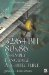# Algebraic Laws Used in This BookAdditive Identity n + 0 = 0 + n = n Multiplicative Identity n1 = 1n = n
 Additive Inverse a b = a + (b)
 Commutative Law of Addition a + b = b + a Commutative Law of Multiplication ab = ba
 Distributive a(b+c) = ab+ac (b+c)/a = b/a + c/a32/64-Bit 80x86 Assembly Language Architecture
ISBN: 1598220020
EAN: 2147483647
Year: 2003
Pages: 191

Similar book on Amazon